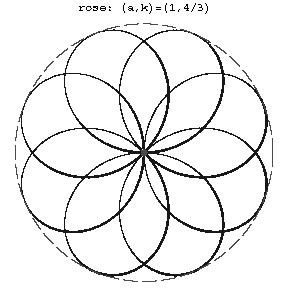# Roses (curves)

Planar curves whose equations in polar coordinates have the form

$$\rho=a\sin k\phi,$$

where $a$ and $k$ are constants. If $k=m/n$ is a rational number, then a rose is an algebraic curve of even order.Figure: r082610a

The order of a rose is equal to $m+n$ if $m$ and $n$ are odd, and to $2(m+n)$ if either $m$ or $n$ is even. The entire curve is situated inside the circle of radius $a$ and consists of congruent parts, called petals (see Fig.). If $k$ is an integer, then the rose consists of $k$ petals for $k$ odd and of $2k$ petals for $k$ even. If $k=m/n$ and $m,n$ are relatively prime, then the rose consists of $m$ petals for $m$ and $n$ odd, and of $2m$ petals when either $m$ or $n$ is even.

When $k$ is irrational there are infinitely many petals. Roses belong to the family of cycloidal curves (cf. Cycloidal curve). They are hypocycloids if $k>1$, and epicycloids if $k<1$.

Roses are also related to the family of cycloidal curves by the fact that they are pedals of epi- and hypocycloids with respect to the centre of their fixed circle.

The arc length of a rose is given by an elliptic integral of the second kind. The area of one petal is $S=\pi a^2/4k$.

Roses are also called curves of Guido Grandi, who was the first to describe them in 1728.